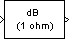# dB Conversion

Convert magnitude data to decibels (dB or dBm)

• Library:
• DSP System Toolbox / Math Functions / Math Operations

•## Description

The dB Conversion block converts a linearly scaled power or amplitude input to dB or dBm. The reference power is 1 Watt for conversions to dB and 1 mWatt for conversions to dBm. The block's Input signal parameter specifies whether the input is a power signal or a voltage signal, and the Convert to parameter controls the scaling of the output. When selected, the Add eps to input to protect against “log(0) = -inf” parameter adds a value of `eps` to all power and voltage inputs. When this parameter is not selected, zero-valued inputs produce `-``Inf` at the output.

## Ports

### Input

expand all

Input signal to be converted, specified as a real scalar, vector, or matrix.

When the Input signal parameter is set to:

• `Power` — The input is treated as a power signal, specified in Watts.

• `Amplitude` — The input is treated as a voltage signal, specified in Volts.

Data Types: `single` | `double`

### Output

expand all

The converted output signal, returned as a scalar, vector, or matrix. The output is the same size, and data type as the input. The output `y` is calculated as follows.

When the Input signal parameter is set to `Power` and Convert to parameter is set to:

• `dB` — The block performs this dB conversion:

```y = 10*log10(u) ```
• `dBm` — The block performs this dBm conversion:

```y = 10*log10(u) + 30 ```

The dBm conversion is equivalent to performing the dB operation after converting the input to milliwatts.

Input u is a real, nonnegative, power signal, specified in Watts.

When the Input signal parameter is set to `Amplitude` and Convert to parameter is set to:

• `dB` — The block performs this dB conversion:

```y = 10*log10(abs(u)^2/R) ```
• `dBm` — The block performs the following dBm conversion:

```y = 10*log10(abs(u)^2/R) + 30 ```

Input u is a real voltage signal, specified in Volts.

The dBm conversion is equivalent to performing the dB operation after converting the `(abs(u)^2/R)` result to milliwatts. The scale factor, R, is specified in Ohms.

Data Types: `single` | `double`

## Parameters

expand all

The logarithmic scaling to which the input is converted, `dB` or `dBm`. The reference power is 1 Watt for conversions to dB and 1 mW for conversions to dBm.

Amplitude conversions use the scale factor specified in ohms by the Load resistance parameter, R, to convert the voltage input to units of power (watts) before converting to dB or dBm.

When the Input signal parameter is set to `Power` and Convert to parameter is set to:

• `dB` — The block performs this dB conversion:

```y = 10*log10(u) ```
• `dBm` — The block performs this dBm conversion:

```y = 10*log10(u) + 30 ```

The dBm conversion is equivalent to performing the dB operation after converting the input to milliwatts.

Input u is a real, nonnegative, power signal, specified in Watts.

Input signal is set to `Amplitude` and Convert to parameter is set to:

• `dB` — The block performs this dB conversion:

```y = 10*log10(abs(u)^2/R) ```
• `dBm` — The block performs this dBm conversion:

```y = 10*log10(abs(u)^2/R) + 30 ```

The dBm conversion is equivalent to performing the dB operation after converting the `(abs(u)^2/R)` result to mW. The scale factor R is specified in Ohms.

Input u is a real voltage signal, specified in Volts.

Tunable: Yes

The type of input signal, `Power` or `Amplitude`.

When Input signal is set to:

• `Power` –– Input u is a real, nonnegative, power signal (units of Watts).

• `Amplitude` –– Input u is a real voltage signal (units of Volts).

The scale factor R, specified in ohms. The block uses the scale factor to convert voltage input to units of power (watts) before converting to dB or dBm.

Tunable: Yes

#### Dependencies

To enable this parameter, set the Input signal parameter to `Amplitude`.

Data Types: `single` | `double` | `int8` | `int16` | `int32` | `uint8` | `uint16` | `uint32` | `Boolean` | `fixed point`
Complex Number Support: Yes

Select this parameter to add `eps` to all input values (power or voltage).

Tunable: Yes

## Block Characteristics

 Data Types `double` | `single` Multidimensional Signals `No` Variable-Size Signals `No`

## Version History

Introduced before R2006a